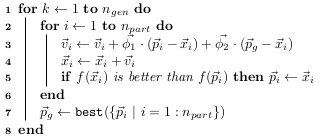# Particle Swarm Optimization¶

This solver is implemented in optunity.solvers.ParticleSwarm. It as available in optunity.make_solver() as ‘particle swarm’.

Particle swarm optimization (PSO) is a heuristic optimization technique. It simulates a set of particles (candidate solutions) that are moving aroud in the search-space [PSO2010], [PSO2002].

In the context of hyperparameter search, the position of a particle represents a set of hyperparameters and its movement is influenced by the goodness of the objective function value.

PSO is an iterative algorithm:

1. Initialization: a set of particles is initialized with random positions and initial velocities. The initialization step is essentially equivalent to Random Search.

2. Iteration: every particle’s position is updated based on its velocity, the particle’s historically best position and the entire swarm’s historical optimum.Particle swarm iterations:

• $$\vec{x}_i$$ is a particle’s position,
• $$\vec{v}_i$$ its velocity,
• $$\vec{p}_i$$ its historically best position,
• $$\vec{p}_g$$ is the swarm’s optimum,
• $$\vec{\phi}_1$$ and $$\vec{\phi}_2$$ are vectors of uniformly sampled values in $$(0, \phi_1)$$ and $$(0, \phi_2)$$, respectively.

PSO has 5 parameters that can be configured (see optunity.solvers.ParticleSwarm):

• num_particles: the number of particles to use
• num_generations: the number of generations (iterations)
• phi1: the impact of each particle’s historical best on its movement
• phi2: the impact of the swarm’s optimum on the movement of each particle
• max_speed: an upper bound for $$\vec{v}_i$$

The number of function evaluations that will be performed is num_particles * num_generations. A high number of particles focuses on global, undirected search (just like Random Search), whereas a high number of generations leads to more localized search since all particles will have time to converge.

Bibliographic references:

 [PSO2010] Kennedy, James. Particle swarm optimization. Encyclopedia of Machine Learning. Springer US, 2010. 760-766.
 [PSO2002] Clerc, Maurice, and James Kennedy. The particle swarm-explosion, stability, and convergence in a multidimensional complex space. Evolutionary Computation, IEEE Transactions on 6.1 (2002): 58-73.